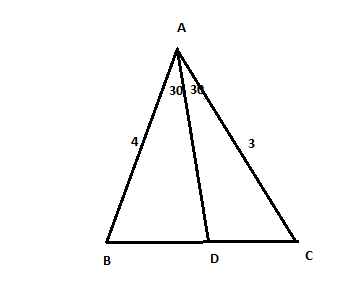Question 9

# In triangle ABC, the internal bisector of $$\angle{A}$$ meets BC at D. If AB = 4, AC = 3 and $$\angle{A}$$ = 60° , then the length of AD is

SolutionBy using cosine rule we can find BC = $$\sqrt{13}$$ . By angle bisector theorem we have BA / BD = AC / DC . Also BD + DC = $$\sqrt{13}$$. So by substitution we get we get BD = 4*$$\sqrt{13}$$/7 . Now using cosine rule in triangle ABD taking AD = x, we get
$$x^2 - 4*\sqrt13*x + 16*(36/49) = 0$$. Solving the equation we get x = $$\frac{12\sqrt 3}{7}$$ .

• All Quant CAT Formulas and shortcuts PDF
• 30+ CAT previous papers with solutions PDF

2 years, 8 months ago

any other method

##### Mridul Tulsyan

1 month, 2 weeks ago

Yes, just use the formula area of triangle= 1/2 x a x b x sin(angle b/w a and b) and then equate the area of 2 smaller triangles to the bigger triangle, it's much faster